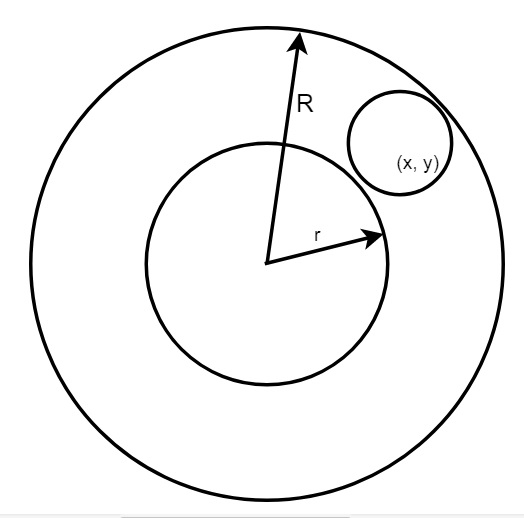# Check if a given circle lies completely inside the ring formed by two concentric circles in C++

We have two circles. The center of both of them is at the origin. The radius of these two circles is given. They are r and R, R > r. Another circle is also present. Its radius (r1) and the center point are given, we have to check whether that point is inside the ring formed by the first two circles or not.We can solve this using the Pythagorean theorem. compute the distance from the center of the circle and origin. Then if (distance – r1) >= r and (distance – r1) <= R, if both are true, then the circle is inside the ring.

## Example

Live Demo

#include <iostream>
#include <cmath>
using namespace std;
bool isInside(int r, int R, int r1, int x, int y) {
int dis = sqrt(x*x+y*y);
return (dis-r1 >= R && dis+r1 <= r);
}
int main() {
int r = 8, R = 4, r1 = 2, x = 6, y = 0;
if (isInside(r, R, r1, x, y))
cout << "Circle is inside the ring." << endl;
else
cout << "Circle is not inside the ring." << endl;
}

## Output

Circle is inside the ring.